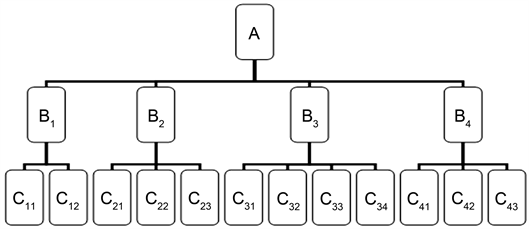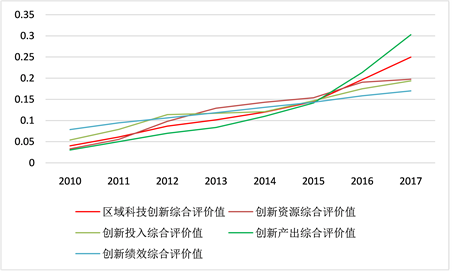# 徐州市区域科技创新体系建设及综合评价研究——基于AHP和熵权法The Construction and Comprehensive Evaluation of Regional Scientific and Technological Innovation System of Xuzhou City——Based on AHP and Entropy Weight Methods

DOI: 10.12677/MM.2019.96087, PDF, HTML, XML, 下载: 365  浏览: 596  科研立项经费支持

Abstract: This paper proposes an evaluation system involving one target layer, four criterion layers and twelve indicators, measuring Xuzhou’s regional scientific and technological innovation capability from 2010 to 2017. The approaches used for determination of weights are combination of subjec-tive weighting and objective weighting methods. Specifically, the weight of the criterion layer is determined by the analytic hierarchy process (AHP) while the weight of the index layer is deter-mined by the entropy weight algorithm. Then, the evaluation values are synthesized in terms of regional scientific and technological innovation capability and indicator layers as well. To conclude, suggestions are recommended in strict accordance with the research results.

1. 引言

2. 区域科技创新评价指标体系Table 1. Evaluation index system of regional science and technology innovation in Xuzhou City

3. 区域科技创新评价模型构建

3.1. 层次分析法(AHP)

1) 建立递阶层次结构模型

2) 构造成对比较判断矩阵

3) 判断矩阵的一致性检查

a) 计算判断矩阵每一行元素的乘积

${M}_{i}=\underset{i=1}{\overset{n}{\prod }}{x}_{ij},\text{\hspace{0.17em}}\text{\hspace{0.17em}}i=1,2,\cdots ,n$

b) 计算 ${M}_{i}$ 的n次方根 ${\stackrel{¯}{W}}_{i}$

${\stackrel{¯}{W}}_{i}=\sqrt[n]{{W}_{i}}$

c) 对向量 $\stackrel{¯}{W}={\left[{\stackrel{¯}{W}}_{1},{\stackrel{¯}{W}}_{2},\cdots ,{\stackrel{¯}{W}}_{n}\right]}^{\text{T}}$ 正规化(归一化处理)

${W}_{i}=\frac{{\stackrel{¯}{W}}_{i}}{\underset{i=1}{\overset{n}{\sum }}{\stackrel{¯}{W}}_{i}}$

$W={\left[{W}_{1},{W}_{2},\cdots ,{W}_{n}\right]}^{\text{T}}$ 即为所求的特征向量。

d) 计算矩阵的最大特征根 ${\lambda }_{\mathrm{max}}$

${\lambda }_{\mathrm{max}}=\underset{i=1}{\overset{n}{\sum }}\frac{{\left(AW\right)}_{i}}{n{W}_{i}}$

e) 为了检验判断矩阵的一致性，需要计算它的一致性指标，

$CI=\frac{{\lambda }_{\mathrm{max}}-n}{n-1}$

$RI=\frac{{{\lambda }^{\prime }}_{\mathrm{max}}-n}{n-1}$

$CR=\frac{CI}{RI}=\frac{{\lambda }_{\mathrm{max}}-n}{RI\cdot \left(n-1\right)}$Table 2. Mean random consistency index

3.2. 熵权算法

1) 形成原始数据矩阵

$X=\left[\begin{array}{cccc}\begin{array}{c}{x}_{11}\\ {x}_{21}\\ ⋮\\ {x}_{m1}\end{array}& \begin{array}{c}{x}_{12}\\ {x}_{22}\\ ⋮\\ {x}_{m2}\end{array}& \begin{array}{c}\cdots \\ \cdots \\ \ddots \\ \cdots \end{array}& \begin{array}{c}{x}_{1n}\\ {x}_{2n}\\ ⋮\\ {x}_{mn}\end{array}\end{array}\right]$ (1)

2) 对原始矩阵进行无量纲化处理

${r}_{ij}={x}_{ij}/{\stackrel{¯}{x}}_{ij}$ (2)

3) 计算第j个指标下，第i个项目的指标值的比重 ${p}_{ij}$

${p}_{ij}={r}_{ij}/\underset{i=1}{\overset{m}{\sum }}{r}_{ij}$ (3)

4) 计算第j个指标的熵值 ${e}_{j}$

${e}_{j}=-k\underset{k=1}{\overset{m}{\sum }}\left({p}_{ij}\cdot \mathrm{ln}{p}_{ij}\right)\text{\hspace{0.17em}}\text{\hspace{0.17em}}\left(j=1,2,\cdots ,n\right)$ (4)

5) 计算第j项指标的差异系数 ${h}_{j}$

${h}_{j}=1-{e}_{j}\text{\hspace{0.17em}}\text{\hspace{0.17em}}\left(j=1,2,\cdots ,n\right)$ (5)

6) 计算第j个指标的熵权 ${w}_{j}$

${w}_{j}=\frac{{h}_{j}}{\underset{i=1}{\overset{n}{\sum }}{h}_{j}}=\frac{1-{e}_{j}}{\underset{i=1}{\overset{n}{\sum }}\left(1-{e}_{j}\right)}\text{\hspace{0.17em}}\text{\hspace{0.17em}}\left(j=1,2,\cdots ,n\right)$ (6)

7) 分别计算各个评价对象的综合评价值：

${u}_{i}=\underset{j=1}{\overset{n}{\sum }}{w}_{j}{p}_{ij}$ (7)

4. 实证研究

4.1. 数据的搜集和整理

4.2. 权重的确定

4.2.1. 准则层权重确定Figure 1. Hierarchical structure diagram

4.2.2. 指标层熵权确定Table 4. Normalized data in the entropy methodTable 5. Entropy value, difference coefficient and entropy weight of each indexTable 6. Total weight of indicator layer

4.3. 综合评价值确定Table 7. Xuzhou regional scientific and technological innovation and comprehensive evaluation value of index layer (2010~2017)Figure 2. Xuzhou regional scientific and technological innovation and comprehensive evaluation value of index layer (2010~2017)

5. 提高徐州市区域科技创新能力的对策建议

5.1. 优化创新环境，建设创新平台

5.2. 增加科技创新投入

5.3. 培育、引进创新创业人才

5.4. 强化政策保障，建立监测和考评机制

  童纪新, 陈继兴, 蔡元成. 基于灰色关联分析的区域科技创新效率评价研究——以江苏省为例[J]. 科技进步与对策, 2011, 28(10): 108-110.  姜文仙. 广东省区域科技创新能力评价研究[J]. 科技管理研究, 2016, 36(8): 75-79+86.  巴吾尔江, 董彦斌, 孙慧, 张其. 基于主成分分析的区域科技创新能力评价[J]. 科技进步与对策, 2012, 29(12): 26-30.  张志新, 孙照吉, 薛翘. 黄河三角洲区域科技创新能力综合分析与评价研究[J]. 经济问题, 2014(4): 100-105.  张燕, 徐燕, 史伟. 基于因子分析法的徐州市农业科技创新能力评价研究[J]. 江苏科技信息, 2019, 36(4): 15-17.  蒋兴华. 区域科技创新能力评价体系构建及综合评价实证研究[J]. 科技管理研究, 2012, 32(14): 64-68.  张立恒. 基于AHP-熵权法的我国区域科技创新可拓学评价模型及实证研究[J]. 工业技术经济, 2019, 38(8): 130-136.  赵黎明, 刘猛. 基于熵权TOPSIS的区域科技创新能力评价模型及实证研究[J]. 天津大学学报(社会科学版), 2014, 16(5): 385-390.  陈艳华. 基于熵权TOPSIS的区域科技创新能力实证研究[J]. 工业技术经济, 2017, 36(5): 46-51.  赵绍娟. 基于DEA模型的徐州市科技创新效率研究[J]. 创新科技, 2019, 19(5): 22-30.